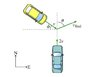# Car Collision at an Angle

• kenau_reveas

#### kenau_reveas

Two cars, both of mass m, collide and stick together. Prior to the collision, one car had been traveling north at speed 2v, while the second was traveling at speed v at an angle phi south of east (as indicated in the figure). After the collision, the two-car system travels at speed v_final at an angle theta east of north.

#### Attachments

•6318.jpg
6.4 KB · Views: 464
Conservation of momentum, remember velocity is a vector quantity.

Show your work so we can determine where you need help.

can anyone help me with the above problem? I've been trying to work it out but i can't find the answer to vfinal... or theta

Like civil_dude said, show your work so we know where you went wrong.

Either use the velocities as vectors or break the conservation of momentum into its x and y components.

i got the other homework problems but i don't think I'm even close for this one. the answer that i have so far for vfinal i have sqrt( (v*cos(phi))^2 + (2v - vsin(phi)^2)

but that doesn't work or feel right either. and i can't even begin to find theta

Are you using energy conservation? The collision is inelastic. Use conservation of momentum.